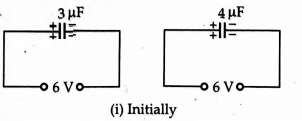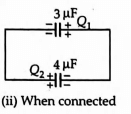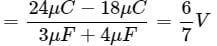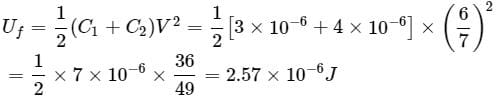Two capacitors, 3μF and 4μF, are individually charged across a 6V6V battery. After being disconnected from the battery, they are connected together with the negative plate of one attached to the positive plate of the other. What is the final total energy stored
• a)
1.26 × 10−4J
• b)
2.57 × 10−4J
• c)
1.25 × 10−6J
• d)
2.57 × 10−6J

### Related TestDiwakar Singh Feb 13, 2022As Q = CV
∴ Initially the charge on each capacitor is
Q1 = C1V1 = (3μF)(6V) = 18μC
and Q2 = C2V2 = (4μF)(6V) = 24μC
When two capacitors is are joined to each other such that negative plate of one is attached with the positive plate of the other. The charges Q1 and Q2 are redistributed till they attain the common potential which is given by.Common potential V = Total Charge/Total capacitanceFinal energy stored,As Q = CV∴ Initially the charge on each capacitor isQ1 = C1V1 = (3μF)(6V) = 18μCand Q2 = C2V2 = (4μF)(6V) = 24μCWhen two capacitors is are joined to each other such that negative plate of one is attached with the positive plate of the other. The charges Q1 and Q2 are redistributed till they attain the common potential which is given by.Common potential V = Total Charge/Total capacitanceFinal energy stored,
Do you know? How Toppers prepare for NEET Exam
With help of the best NEET teachers & toppers, We have prepared a guide for student who are preparing for NEET : 15 Steps to clear NEET Exam